### Home > A2C > Chapter 8 > Lesson 8.2.3 > Problem8-146

8-146.

Match each equation with the appropriate graph. Do this without using a graphing calculator.1. $y=\sin\left(x+\frac{\pi}{2}\right)$

1. $y = \sin\left(2x\right)$

1. $y=2\sin\left(\frac{x}{2}\right)$

1. $y = \sin\left(x\right) − 3$

1. $y = −\sin[2(x − \frac { \pi } { 8 })]$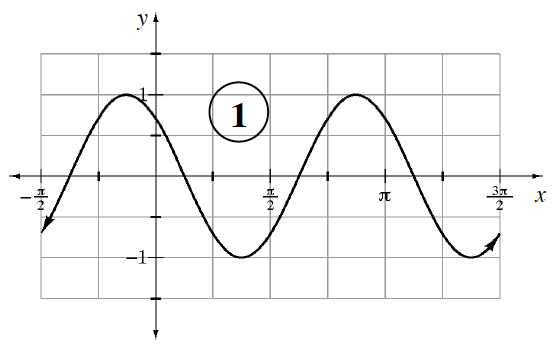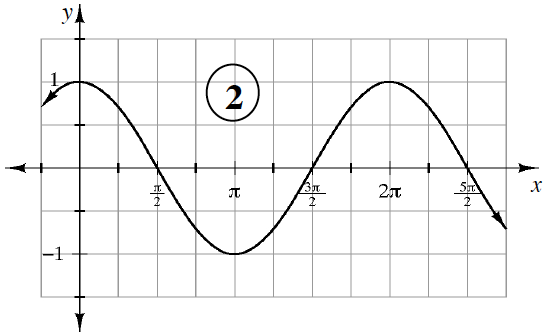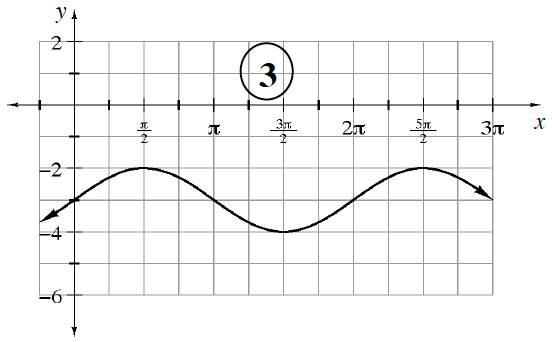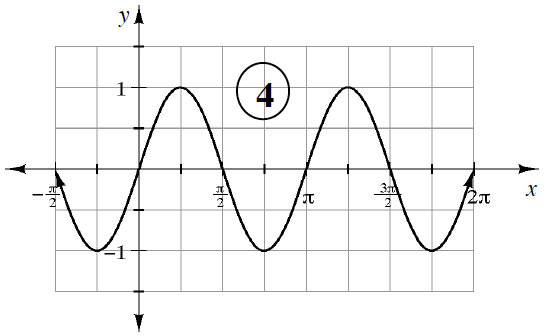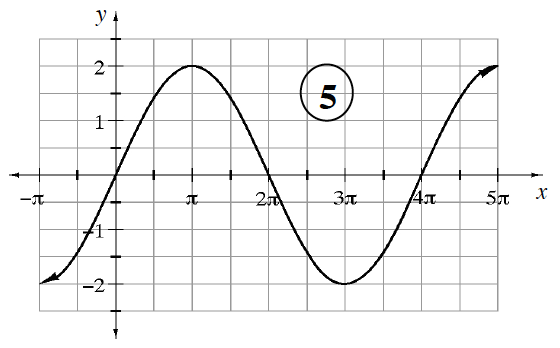(a) $2$
(b) $4$
(c) $5$
(d) $3$
(e) $1$